# AP Board 7th Class Maths Solutions Chapter 14 Understanding 3D and 2D Shapes InText Questions

AP State Syllabus AP Board 7th Class Maths Solutions Chapter 14 Understanding 3D and 2D Shapes InText Questions and Answers.

## AP State Syllabus 7th Class Maths Solutions 14th Lesson Understanding 3D and 2D Shapes InText QuestionsQuestion 1.
Estimate the number of cubes in the following arrangement. (Page No. 273)
Solution: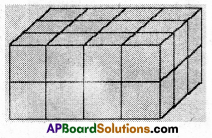4 × 3 × 2 = 24 No. of cubes in the above fig. = 24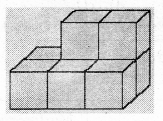3 × 2 + 2 = 6 + 2 = 8
No. of cubes in the above fig. = 8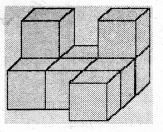3 + 2 + 2 + 1 + 1 = 9
No. of cubes in the above fig. = 9Question 2.
Two dice are placed side by side as shown. Can you say what the total would be on the faces opposite to them. (Page No. 274)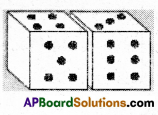i) 5 + 6 ii) 4 + 3
Solution:
i) Sum of the opposite faces = 2 + 1 = 3
ii) Sum of the opposite faces = 3 + 4 = 7

Question 3.
Three cubes each with 2 cm are placed side by side to form a cuboid. Try to make an oblique sketch and say what could be its length, breadth and height. (Page No. 274)
Solution: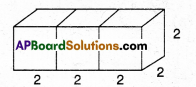Length =2 + 2 + 2 = 6cm
Height = 2 cmDo This

Question 1.
Make clay (or plasticine) models of the following solids and make vertical or horizontal cuts. Draw rough sketches of the cross-sections you obtain. Name them if possible. (Page No. 274)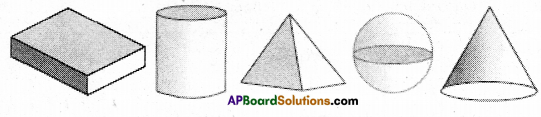Solution: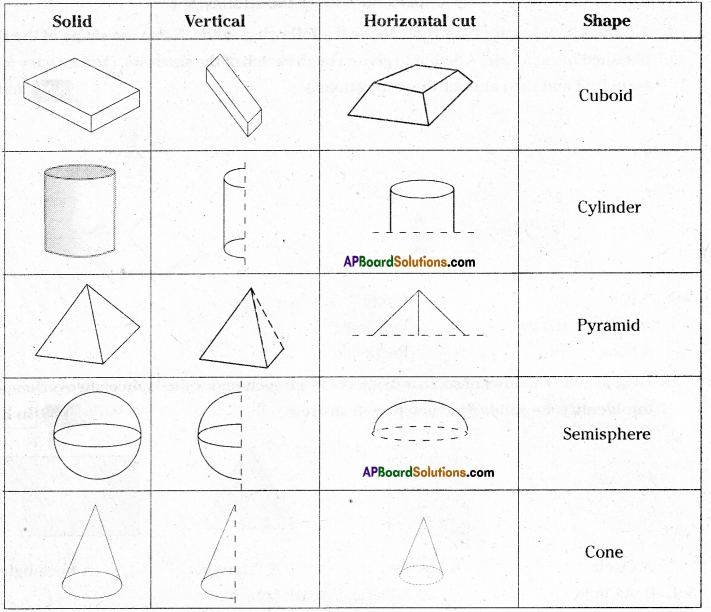Question 2.
What cross-section do you get when you give a i) vertical cut ii) horizontal cut to the following solids. (Page No. 275)
a) A brick
b) A round apple
c) A die
d) A circular pipe
e) An ice-cream cone
Solution:

 Solid Vertical cut Horizontal cut Brick Square/rectangle Rectangle A round apple Circle Circle Die Square Square Circular pipe Rectangle Circle ice-cream cone Triangle Circle